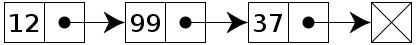### The WordPress Theme Frameworks - ultimate guide for starter

WordPress is one of the magnificent open source web software that you can use to create a beautiful website or blog. In the beginning days of WordPress, there were some horrible problems with the way how themes were developed and maintained. There was no good way of upgrading WordPress themes without losing all the custom styling options. There was no way to prevent copying andWordPress Logo
pasting of the same functionality code in all themes. But now, Creating custom WordPress themes is exceptionally instinctive and relatively easy using WordPress application programming interface(API).

If you’re going to design a WordPress site, there are a few ways you can start :

## A differential equation (D.E.) of the form dy/dx= f (x, y) and an initial condition (x0,y0), we would like to a find function y = f (x) that satisfies the D.E. and passes through the point (x0,y0). In some cases, one can identify the general solution to the D.E. by inspection or using methods such as separation of variables and integration. But sometimes, either we don’t have the analytic tools in our tool bag to solve the D.E. or an analytic solution is not possible.That’s when we need a numerical method for solving the D.E. One such method is Euler’s Method.

### Longest Common Sub-sequence (LCS)

In computer science we use dynamic programming for solving complex problem. A good example for dynamic programming is longest common subsequence.
Dynamic programming is a method for solving a complex problem by breaking it down into a collection of simpler subproblems. It is applicable to problems exhibiting the properties of overlapping subproblems and optimal substructure. Sometimes the subproblems are not independent, that is when sub-problems share subsubproblems. Dynamic programming algorithm solves every sub-problem just once and then saves its answer in a table.

## Fibonacci implementation

The Fibonacci Sequence is the series of numbers: 0, 1, 1, 2, 3, 5, 8, 13, 21, 34, ... The next number is found by adding up the two numbers before it. The 2 is found by adding the two numbers
before it (1+1) Similarly, the 3 is found by adding the two numbers before it (1+2), And the 5 is (2+3), and so on! The first two numbers in the Fibonacci sequence are either 1 and 1, or 0 and 1, depending on the chosen starting point of the sequence, and each subsequent number is the sum of the previous two. It is an interesting series and we all know it very well. But how many of us use it in our practical life??? Now try to solve a problem by implementing fibonacci series..
Let's see some easy ACM problems.

Happy coding...

### Some UVa easy problems for beginner.

#### Beginner  should start from simple problems. Here is some UVa easy problems for beginer.

1. 11172:Relational Operator.

2. 10071 - Back To High School Physics

3. 10055 - Hashmat the Brave Warrior

## How to Add two big number using string:

It's easy to add two numbers in any programming language. For example, if int a = 2; int b = 3, then we just add a + b . Now if we try to add two numbers each having 100 digits like 10000000000000000000000000000000000...., what should we do ?

There is no data type to hold 100 digit in a variable. So what can we do ? The process is to take input as string. Then we can add those numbers.
For example:

### Pattern 6:

#### Discussion:

Reverse form of pattern 2. Using scanf() function to take user input.

### Pattern 5:

#### Discussion:

Reverse form of pattern pattern 1. It's take user input to print stars.

### Pattern 4:

#### Discussion:

It print stars like reverse pyramid. Reverse form of pattern 3.

### Pattern 3:

Discussion:
This code print stars like Pyramid. This is user input code using scanf().

### Pattern 1:


Discussion: 
This code will print 1 star in first row and from next row it increase
by 1 to n.

### Pattern 2:

#### Discussion:

This is the reverse form of pattern 1.The following code print stars so that, the first
row prints as length user enter. The number of stars decrease by one in every new row.
This code ask user to enter choice using scanf().

# How to print stars pattern ( * ) in C programming ?

We can play with * and print them in many ways, many style. Let's see some awesome pattern of stars and try to do this by computer.
At first try yourself, then go to see code.

Pattern 1:

#### Pattern 6:

Code for this above pattern.

#### Pattern 7:

Code for this above pattern.

#### Pattern 8:

Code for this above pattern.

#### Try print star in java programming.

Happy coding...UVa Online Judge

## Art of programming contest is a pdf e book which containing c programming, data structure and algorithm for UVa online judge user.acm icpcLinked List operation